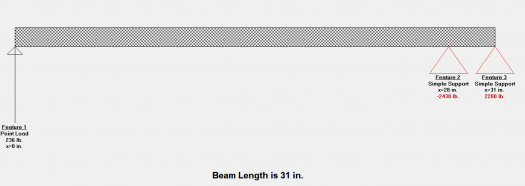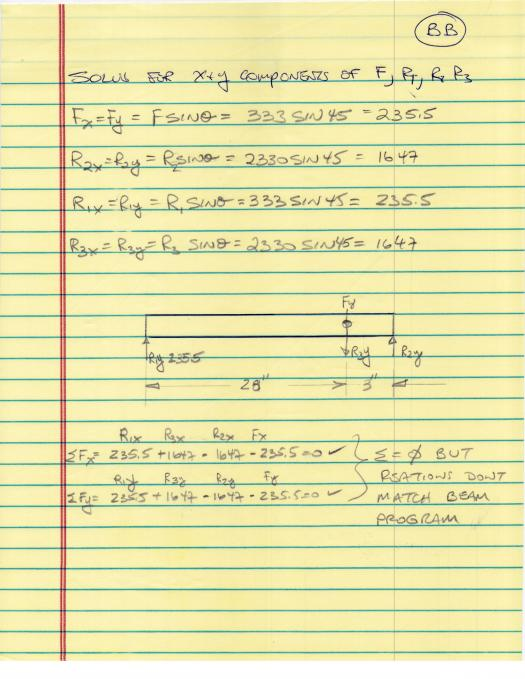# What am I missing when I rotate a tripod leg 45 degrees to solve for loads

2 posts / 0 new
JAG-E
What am I missing when I rotate a tripod leg 45 degrees to solve for loads

I solved for the loads on a tripod leg that creates a 45 degree angle with the ground. The leg is 2x2" tube. The legs fold up and then swings into open position and stopped by R2. After solving for the loads I rotated the leg to the horizontal position. I solved for the X&Y components. This allowed me to use a beam program with the Y loads and reactions to determine the moment diagram and bending stress. I would then solve for the axial stresses using the X components. I could then combine the bending stress and axial stresses. That was the plan and I thought a good check on my math.

When I place the rotated leg into the beam program and apply a load (R1Y=235.5= Feature 1) to one end which is the Y component of the reaction with the ground, I expected the reactions at the two supports (Pivot and R2 stop) to equal the calculated values R2Y =1647 & (Fy+R3Y=1882). They don't! I thought I may have the signs wrong so I tried changing signs but could never get the calculated loads in the beam program.

Attached are two sheets for calculations and one from the beam program. The R1Y is the only force I used in the beam program. Expecting Feature 3 = R2Y = 1647 from the hand calculations to be the same end reaction, and similar for Feature 2.

Can someone identify the hole in my logic or calculation?
I hope the 3 images are viewable.Jhun VertI did not see the load Fy in the beam program. The only load you apply is the R1y. I manually check the calculations of the beam program for R1y only load, I arrive the same answer of 2433.5 lb as indicated in your screenshot.

Have you tried including the Fy applied downward at 28" from left?

Subscribe to MATHalino on